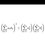# Ten words (Just for fun)

How well can we summarise proofs?

Come up with proofs for the following statements in ten words or less. Who can make the most rigorous, accurate proof in just ten words?

$1$. Among $51$ integers from $1$ to $100$ inclusive, there exists two summing to $101$.

$2$. $8^{n}-5^{n}$ is a multiple of $3$ where $n$ is a positive integer.

$3$. The point $O$ in equilateral triangle $ABC$ such that $OA+OB+OC$ is minimised is the center of $ABC$.

All operations, except for brackets, are words.Note by Joel Tan
5 years ago

This discussion board is a place to discuss our Daily Challenges and the math and science related to those challenges. Explanations are more than just a solution — they should explain the steps and thinking strategies that you used to obtain the solution. Comments should further the discussion of math and science.

When posting on Brilliant:

• Use the emojis to react to an explanation, whether you're congratulating a job well done , or just really confused .
• Ask specific questions about the challenge or the steps in somebody's explanation. Well-posed questions can add a lot to the discussion, but posting "I don't understand!" doesn't help anyone.
• Try to contribute something new to the discussion, whether it is an extension, generalization or other idea related to the challenge.
• Stay on topic — we're all here to learn more about math and science, not to hear about your favorite get-rich-quick scheme or current world events.

MarkdownAppears as
*italics* or _italics_ italics
**bold** or __bold__ bold
- bulleted- list
• bulleted
• list
1. numbered2. list
1. numbered
2. list
Note: you must add a full line of space before and after lists for them to show up correctly
paragraph 1paragraph 2

paragraph 1

paragraph 2

[example link](https://brilliant.org)example link
> This is a quote
This is a quote
    # I indented these lines
# 4 spaces, and now they show
# up as a code block.

print "hello world"
# I indented these lines
# 4 spaces, and now they show
# up as a code block.

print "hello world"
MathAppears as
Remember to wrap math in $$ ... $$ or $ ... $ to ensure proper formatting.
2 \times 3 $2 \times 3$
2^{34} $2^{34}$
a_{i-1} $a_{i-1}$
\frac{2}{3} $\frac{2}{3}$
\sqrt{2} $\sqrt{2}$
\sum_{i=1}^3 $\sum_{i=1}^3$
\sin \theta $\sin \theta$
\boxed{123} $\boxed{123}$

Sort by:

2) If N>=0, 8^N - 5^N = 0 (mod 3)

- 4 years, 11 months ago

There can be 49 pairs summing to 101

- 5 years ago

Q.2 It has one factor(8-5) = 3 means it is divisible by 3 Q.3 O is crcumcentre

- 5 years ago

Maximum is at the fermat point, not at the circumcentre, although it is true in this case since it is equilateral.

- 4 years, 12 months ago

2.8^n-5^=(8-5)k

- 5 years ago

1. Prove that sum of two integers within 100 is 101.

- 5 years ago

Proofs:

1) Gauss' pairing trick paired with pigeonhole principle.

2) $=$ GP with ends $5^{n-1}, 8^{n-1}$, ratio $1.6$, summed thrice.

3) By construction of Fermat point, $O = X(13)$.

1) Alliteration 'p's yay! No I really did not want to refer to any language stuff.

2) It is trivial to see that all terms of the GP are integers, and ends refer to initial and final terms. I would have preferred $3\sum^{n-1}_{k=0}8^{n-1-k}5^k$ but I am not sure how many words would that be.

3) Sorry for the overkill :P But anyway I could have mentioned an equilateral triangle is acute for clarity.

By the way, nice choice of problems!

- 5 years ago

$8^{n}-5^{n}=8^{n}-(8-3)^{n}=3A$

- 5 years ago

1) There are $50$ pairs with sum $101$. PHP.

- 5 years ago

2) $8^n - 5^n \equiv 2^n - 2^n = 0 \pmod{3}$

- 5 years ago

Isn't it supposed to be $8^{n}-5^{n}$ in the very first step...??

- 5 years ago

Yes, typoed it.

- 5 years ago